Warning

This documents an unmaintained version of NetworkX. Please upgrade to a maintained version and see the current NetworkX documentation.

# shortest_augmenting_path¶

shortest_augmenting_path(G, s, t, capacity='capacity', residual=None, value_only=False, two_phase=False, cutoff=None)[source]

Find a maximum single-commodity flow using the shortest augmenting path algorithm.

This function returns the residual network resulting after computing the maximum flow. See below for details about the conventions NetworkX uses for defining residual networks.

This algorithm has a running time of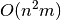for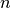nodes and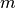edges.

Parameters: G (NetworkX graph) – Edges of the graph are expected to have an attribute called ‘capacity’. If this attribute is not present, the edge is considered to have infinite capacity. s (node) – Source node for the flow. t (node) – Sink node for the flow. capacity (string) – Edges of the graph G are expected to have an attribute capacity that indicates how much flow the edge can support. If this attribute is not present, the edge is considered to have infinite capacity. Default value: ‘capacity’. residual (NetworkX graph) – Residual network on which the algorithm is to be executed. If None, a new residual network is created. Default value: None. value_only (bool) – If True compute only the value of the maximum flow. This parameter will be ignored by this algorithm because it is not applicable. two_phase (bool) – If True, a two-phase variant is used. The two-phase variant improves the running time on unit-capacity networks from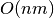to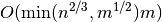. Default value: False. cutoff (integer, float) – If specified, the algorithm will terminate when the flow value reaches or exceeds the cutoff. In this case, it may be unable to immediately determine a minimum cut. Default value: None. R – Residual network after computing the maximum flow. NetworkX DiGraph NetworkXError – The algorithm does not support MultiGraph and MultiDiGraph. If the input graph is an instance of one of these two classes, a NetworkXError is raised. NetworkXUnbounded – If the graph has a path of infinite capacity, the value of a feasible flow on the graph is unbounded above and the function raises a NetworkXUnbounded.

Notes

The residual network R from an input graph G has the same nodes as G. R is a DiGraph that contains a pair of edges (u, v) and (v, u) iff (u, v) is not a self-loop, and at least one of (u, v) and (v, u) exists in G.

For each edge (u, v) in R, R[u][v]['capacity'] is equal to the capacity of (u, v) in G if it exists in G or zero otherwise. If the capacity is infinite, R[u][v]['capacity'] will have a high arbitrary finite value that does not affect the solution of the problem. This value is stored in R.graph['inf']. For each edge (u, v) in R, R[u][v]['flow'] represents the flow function of (u, v) and satisfies R[u][v]['flow'] == -R[v][u]['flow'].

The flow value, defined as the total flow into t, the sink, is stored in R.graph['flow_value']. If cutoff is not specified, reachability to t using only edges (u, v) such that R[u][v]['flow'] < R[u][v]['capacity'] induces a minimum s-t cut.

Examples

>>> import networkx as nx
>>> from networkx.algorithms.flow import shortest_augmenting_path


The functions that implement flow algorithms and output a residual network, such as this one, are not imported to the base NetworkX namespace, so you have to explicitly import them from the flow package.

>>> G = nx.DiGraph()